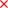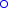## Tic Tac Toe Java Applet

Tic is a Java Applet that plays the game Tic Tac Toe: Two players alternately placees ands on a 3x3 board, the one who first gets three of his symbols in a line (horizontal, vertical or diagonal) wins. You start with!

The computer's playing algorithm works as follows:

• The computer internally carries out all possible moves and rates the resulting situations assuming that both players always choose the best move.
• If the best rating is taken by several moves, these moves are rated assuming that the computer always chooses the best move while the human player chooses every possible move with equal probability.
• If still several best moves remain, the computer chooses one at random.

"Rating assuming that both players always choose the best move" given a board situation and whose turn it is is recursively done as follows:

• If in the rated situationhas won, return 1.
• Ifhas won, return -1.
• Else, internally carry out all possible moves, rate the resulting situations recursively using this algorithm and return the best of the ratings (seen from whose turn it is, i.e. maximum if it's's turn and minimum if it's's turn)

"Rating assuming that the computer always chooses the best move while the human player chooses every possible move with equal probability" given a board situation and whose turn it is is recursively done as follows:

• If it's the computer's turn, rate using the first algorithm.
• Ifhas won, return 1.
• Ifhas won, return -1.
• Else, internally carry out all possible moves, rate the resulting situations recursively using this algorithm and return the average of the ratings.

Because this approach is exhaustive, i.e. searches the whole tree of all possible games, it always returns the really best move. This is possible because Tic Tac Toe is such a simple game - although even in this simple game (not taking into accout that many games end before the board is full) 9! = 362 880 different games are possible and the game tree consists of 986 400 nodes!

The source: